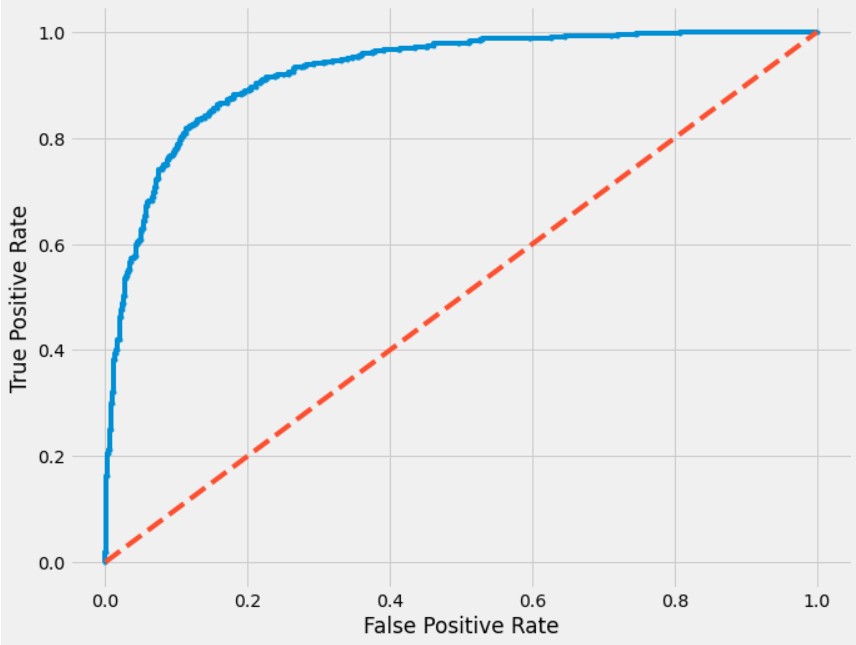# The Beginners’ Guide to the ROC Curve and AUC

Original article was published by Chetan Ambi on Artificial Intelligence on MediumIn the previous article here, you have understood classification evaluation metrics such as Accuracy, Precision, Recall, F1-Score, etc. In this article, we will go through another important evaluation metric AUC-ROC score.

# What is AUC-ROC

ROC curve (Receiver Operating Characteristic curve) is a graph showing the performance of a classification model at different probability thresholds.

ROC graph is created by plotting FPR Vs. TPR where FPR (False Positive Rate) is plotted on the x-axis and TPR (True Positive Rate) is plotted on the y-axis for different probability threshold values ranging from 0.0 to 1.0.

True Positive Rate (TPR) refers to the ratio of correctly predicted positive labels from all the positive labels.

False Positive Rate (FPR) refers to the ratio of incorrectly predicted positive labels from all the negative labels.

AUC stands for Area under the ROC Curve. It measures the entire two-dimensional area underneath the entire ROC curve from (0,0) to (1,1).

A typical AUC-ROC looks like as below —

# Why AUC-ROC

It will be more flexible if we can predict the class label probabilities instead of predicting the class labels itself. Because using the class probabilities we can calibrate probability threshold values. For example, in Logistic Regression, by default 0.5 is considered as a probability threshold. Anything in the range [0.0 — 0.49] is a negative label and anything in the range [0.5–1.0] is the positive label. We can modify this probability threshold of 0.5 and may get better results. Similarly, with AUC ROC, we plot FPR Vs. TPR by using different probability thresholds and we can choose the best performing the threshold based on domain knowledge and other factors.

# How AUC-ROC works

Let’s try to understand how AUC ROC works with an example. Consider below toy data where `y` is the actual class label and `y_pred` is the predicted probability. The rest of the columns are predicted probabilities at different thresholds. For each of the threshold, the corresponding FPR and TPR is calculated and mentioned in the below image. For example, FPR and TPR for threshold `y_pred(0.2)` is `(0.83333, 1)` and so on.

Note: In this example, I have used only 6 thresholds however, one can try using any number thresholds. More the number of thresholds smoother the ROC curve is.

If we were to plot these points, we will get the ROC curve as below. The entire area under the blue curve is AUC (Area Under ROC Curve).

You can find the sample code below on how to draw the ROC curve and how to calculate AUC ROC.